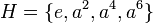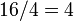Center of M16

This article is about a particular subgroup in a group, up to equivalence of subgroups (i.e., an isomorphism of groups that induces the corresponding isomorphism of subgroups). The subgroup is (up to isomorphism) cyclic group:Z4 and the group is (up to isomorphism) M16 (see subgroup structure of M16).
The subgroup is a normal subgroup and the quotient group is isomorphic to Klein four-group.
VIEW: Group-subgroup pairs with the same subgroup part | Group-subgroup pairs with the same group part| Group-subgroup pairs with the same quotient part | All pages on particular subgroups in groups

Definition

We consider the group:$G = M_{16} = \langle a,x \mid a^8 = x^2 = e, xax = a^5 \rangle$

with$e$ denoting the identity element.

This is a group of order 16, with elements:$\! \{ e, a, a^2, a^3, a^4, a^5, a^6, a^7, x, ax, a^2x, a^3x, a^4x, a^5x, a^6x, a^7x \}$

We are interested in the subgroup:$\! H = \{ e, a^2, a^4, a^6 \}$

This subgroup is isomorphic to cyclic group:Z4. It is a normal subgroup and the quotient group is isomorphic to the Klein four-group.

Here is the multiplication table for$H$. Note that$H$ is an abelian group so we don't have to worry about left/right issues:

Element/element$e$$a^2$$a^4$$a^6$$e$$e$$a^2$$a^4$$a^6$$a^2$$a^2$$a^4$$a^6$$e$$a^4$$a^4$$a^6$$e$$a^2$$a^6$$a^6$$e$$a^2$$a^4$

Cosets

The subgroup is normal, so its left cosets coincide with its right cosets. Since it has order four and index$16/4 = 4$, there are four cosets:$\! \{ e, a^2, a^4, a^6 \}, \{ a, a^3, a^5, a^7 \}, \{ x, a^2x, a^4x, a^6x \}, \{ ax, a^3x, a^5x, a^7x \}$

The quotient group is isomorphic to a Klein four-group, and the multiplication table on cosets is given below. Note that the group is an abelian group, so we don't have to worry about left/right issues:

Element/element$\{ e, a^2, a^4, a^6 \}$$\{ a, a^3, a^5, a^7 \}$$\{ x, a^2x, a^4x, a^6x \}$$\{ ax, a^3x, a^5x, a^7x \}$$\{ e, a^2, a^4, a^6 \}$$\{ e, a^2, a^4, a^6 \}$$\{ a, a^3, a^5, a^7 \}$$\{ x, a^2x, a^4x, a^6x \}$$\{ ax, a^3x, a^5x, a^7x \}$$\{ a, a^3, a^5, a^7 \}$$\{ a, a^3, a^5, a^7 \}$$\{ e, a^2, a^4, a^6 \}$$\{ ax, a^3x, a^5x, a^7x \}$$\{ x, a^2x, a^4x, a^6x \}$$\{ x, a^2x, a^4x, a^6x \}$$\{ x, a^2x, a^4x, a^6x \}$$\{ ax, a^3x, a^5x, a^7x \}$$\{ e, a^2, a^4, a^6 \}$$\{ a, a^3, a^5, a^7 \}$$\{ ax, a^3x, a^5x, a^7x \}$$\{ ax, a^3x, a^5x, a^7x \}$$\{ x, a^2x, a^4x, a^6x \}$$\{ a, a^3, a^5, a^7 \}$$\{ e, a^2, a^4, a^6 \}$

Complements

The subgroup has no permutable complements. Since it is a normal subgroup, this also means it has no lattice complements.

Properties related to complementation

Property Meaning Satisfied? Explanation Comment
complemented normal subgroup normal subgroup with permutable complement No see above
permutably complemented subgroup subgroup with permutable complement No
lattice-complemented subgroup subgroup with lattice complement No
retract has a normal complement No
direct factor normal subgroup with normal complement No

Arithmetic functions

Function Value Explanation
order of whole group 16
order of subgroup 4
index 4
size of conjugacy class 1
number of conjugacy classes in automorphism class 1

Effect of subgroup operators

Function Value as subgroup (descriptive) Value as subgroup (link) Value as group
normalizer whole group$\langle a,x \rangle$ M16
centralizer whole group$\langle a,x \rangle$ M16
normal core the subgroup itself current page cyclic group:Z4
normal closure the subgroup itself current page cyclic group:Z4
characteristic core the subgroup itself current page cyclic group:Z4
characteristic closure the subgroup itself current page cyclic group:Z4
commutator with whole group trivial subgroup -- trivial group

Subgroup-defining functions

The subgroup is a characteristic subgroup of the whole group and arises as a result of many subgroup-defining functions on the whole group. Some of these are given below.

Subgroup-defining function Meaning in general Why it takes this value
center elements that commute with every element of the group Follows from multiplication table
ZJ-subgroup center of the join of abelian subgroups of maximum order The join of abelian subgroups of maximum order is the whole group, so its center is the group's center.
first agemo subgroup in a finite$p$-group, subgroup generated by all$p^{th}$ powers. Here$p = 2$ This is precisely the set of squares in the group.

Subgroup properties

Invariance under automorphisms and endomorphisms: basic properties

Property Meaning Satisfied? Explanation
normal subgroup invariant under inner automorphisms Yes Follows from being the first agemo subgroup.
characteristic subgroup invariant under all automorphisms Yes Follows from being the first agemo subgroup.
fully invariant subgroup invariant under all endomorphisms Yes Follows from being the first agemo subgroup.

Word expression related properties and corollaries about invariance under automorphisms and endomorphisms

Property Meaning Satisfied? Explanation
marginal subgroup (complicated) Yes Follows from being the center. Center is marginal.
finite direct power-closed characteristic subgroup any finite direct power is closed in the corresponding direct power of the center Yes center is finite direct power-closed characteristic, fully invariant implies finite direct power-closed characteristic
verbal subgroup generated by set of words Yes Follows from being the first agemo subgroup.
image-closed characteristic subgroup image under any surjective homomorphism from whole group is characteristic in target group Yes Follows from its being a verbal subgroup.
image-closed fully invariant subgroup image under any surjective homomorphism from whole group is fully invariant in target group Yes Follows from its being a verbal subgroup.

Resemblance-based properties and corollaries about invariance under automorphisms and endomorphisms

Property Meaning Satisfied? Explanation
isomorph-free subgroup no other isomorphic subgroup No$\{e,a^2x,a^4,a^6x \}$ (see non-central Z4 in M16) is an isomorphic subgroup.
intermediately characteristic subgroup characteristic in every intermediate subgroup No In the intermediate subgroup$\langle a^2, x \rangle$, which is direct product of Z4 and Z2 in M16, the subgroup is not characteristic.
isomorph-normal subgroup every isomorphic subgroup is normal Yes The only other isomorphic subgroup is$\{e,a^2x,a^4,a^6x \}$ (see non-central Z4 in M16) and this is normal.
isomorph-characteristic subgroup every isomorphic subgroup is characteristic Yes The only other isomorphic subgroup is$\{e,a^2x,a^4,a^6x \}$ (see non-central Z4 in M16) and this is characteristic.

Invariance under 1-automorphisms and 1-endomorphisms

Property Meaning Satisfied? Explanation
1-endomorphism-invariant subgroup invariant under all 1-endomorphisms of the group Yes Follows from its being precisely the set of squares.
1-automorphism-invariant subgroup invariant under all 1-automorphisms of the group Yes Follows from being 1-endomorphism-invariant.
quasiautomorphism-invariant subgroup invariant under all quasiautomorphisms Yes Follows from being 1-automorphism-invariant

Cohomology interpretation

We can think of$G$ as an extension with abelian normal subgroup$H$ and quotient group$G/H$. Since$H$ is in fact the center, the action of the quotient group on the normal subgroup is the trivial group action. We can thus study$G$ as an extension group arising from a cohomology class for the trivial group action of$G/H$ (which is a Klein four-group) on$H$ (which is cyclic group:Z4).

GAP implementation

The group and subgroup can be constructed using GAP's SmallGroup and Center functions as follows:

G := SmallGroup(16,6); H := Center(G);

The GAP display looks as follows:

gap> G := SmallGroup(16,6); H := Center(G);
<pc group of size 16 with 4 generators>
Group([ f3, f4 ])